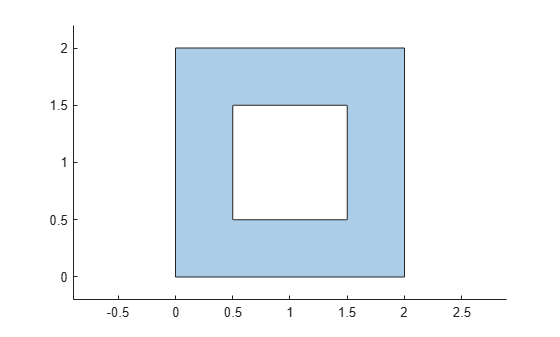# perimeter

Perimeter of `polyshape`

## Syntax

``P = perimeter(polyin)``
``P = perimeter(polyin,I)``

## Description

example

````P = perimeter(polyin)` returns the perimeter of a `polyshape` object, which is the sum of the lengths of its boundaries.```
````P = perimeter(polyin,I)` returns the perimeter of the `I`th boundary of `polyin`.This syntax is only supported when `polyin` is a scalar `polyshape` object.```

## Examples

collapse all

Create a polygon with one hole, and compute its perimeter.

```V = [0 0; 0 2; 2 2; 2 0; NaN NaN; 0.5 0.5; 0.5 1.5; 1.5 1.5; 1.5 0.5]; polyin = polyshape(V); plot(polyin) axis equal````P = perimeter(polyin)`
```P = 12 ```

## Input Arguments

collapse all

Input `polyshape`, specified as a scalar, vector, matrix, or multidimensional array.

Data Types: `polyshape`

Boundary index, specified as a scalar integer or vector of integers. Each element of `I` corresponds to a single boundary of the input `polyshape`.

Data Types: `double` | `single` | `int8` | `int16` | `int32` | `int64` | `uint8` | `uint16` | `uint32` | `uint64`